Get instant live expert help with Excel or Google Sheets“My Excelchat expert helped me in less than 20 minutes, saving me what would have been 5 hours of work!”

#### Post your problem and you'll get expert help in seconds

Your message must be at least 40 characters
Our professional experts are available now. Your privacy is guaranteed.

# Excel MINUTE Function

While working with Excel, we are able to determine the value for minutes of any given time by using the MINUTE function.  This step by step tutorial will assist all levels of Excel users in the usage and syntax of the MINUTE function.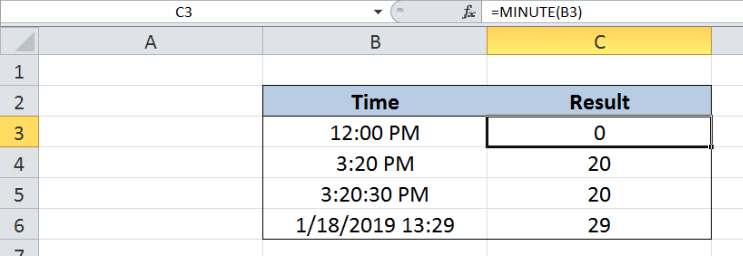Figure 1. Final result: Excel MINUTE function

## Syntax of the MINUTE Function

MINUTE returns an integer that represents the value for minutes of any given time;value ranges from 0 to 59

`=MINUTE(serial_number)`

• serial_number  – the time whose minutes value we want to find; time may be entered as decimal numbers, text strings within quotation marks, formulas or cell reference to a value of time
• The argument serial_number may also be a value representing a combination of date and time; time values are represented by a decimal number corresponding to its portion relative to one day (for example, 6:00 AM is represented as 0.25, since it is 1/4 of a day)

## Setting up our Data

Our data consists of two columns: Time (column B) and Result (column C).  We want to extract the minute component of the time in column B. The results will be recorded in column C.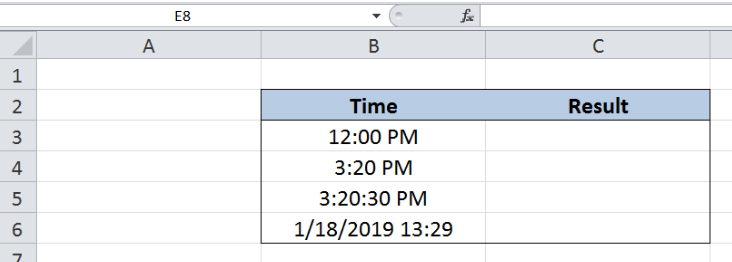Figure 2. Sample data to extract the minute component of time

## Extract the minute component of time in hh:mm format

In order to determine the value for minutes of any given time by using the MINUTE function, let us follow these steps:

Step 1.  Select cell C3

Step 2.  Enter the formula: `=MINUTE(B3)`

Step 3.  Press ENTER

As a result, the value in cell C3 is “0”, because the minute component of the time 12:00 PM in B3 is zero “0”.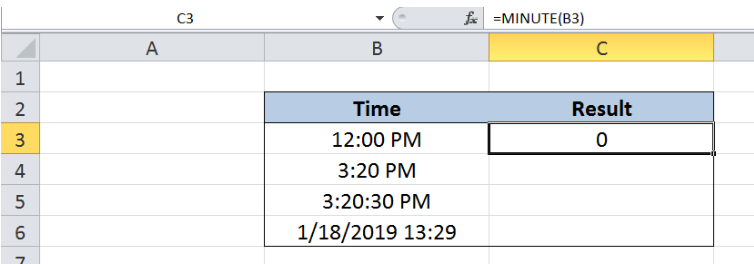Figure 3.  Using MINUTE function to extract the minute component of time

In the same way, when we enter a different time as the argument in our MINUTE function, we will obtain the minute component only.  Let us take another example:

Step 1.  Select cell C4

Step 2.  Enter the formula: `=MINUTE(B4)`

Step 3.  Press ENTER

As a result, the value in cell C4 is “20”, because the minute component of  3:20 PM in B4 is  “20”.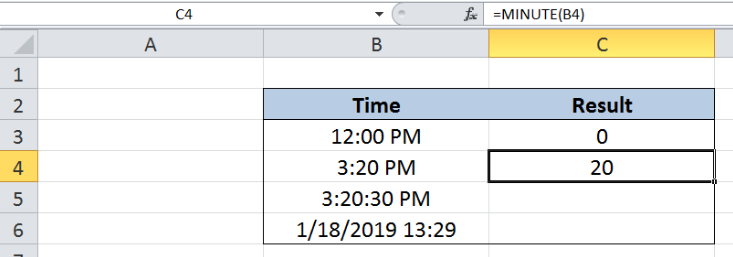Figure 4.  MINUTE function extracting the minute component of time in hh:mm format

## Extract the minute component of time in hh:mm:ss format

The procedure for extracting the minute component of time in a format which includes the seconds value is the same as in our previous example.  Let us follow these steps:

Step 1.  Select cell C5

Step 2.  Enter the formula: `=MINUTE(B5)`

Step 3.  Press ENTER

Note that the time in B5, “3:20:30 PM”, has a seconds value of 30, but the result in cell C5 is still “20”, which is the same as in cell C4.  The MINUTE function only extracts the minute component of a given time, regardless of the hour or seconds value.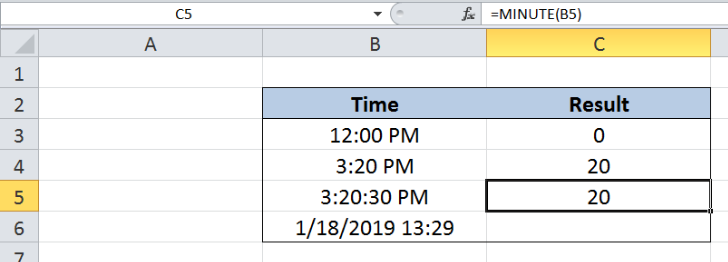Figure 5.  MINUTE function extracting the minute component of time in hh:mm:ss format

## Extract the minute component given a date and time

The MINUTE function is still able to extract the minute component of time, even when the argument is a combination of date and time. Let us follow these steps:

Step 1.  Select cell C6

Step 2.  Enter the formula: `=MINUTE(B6)`

Step 3.  Press ENTER

MINUTE function completely disregards other components and returns only the value for minutes.  As a result, the value in cell C6 is “29”, from the given time of 13:29.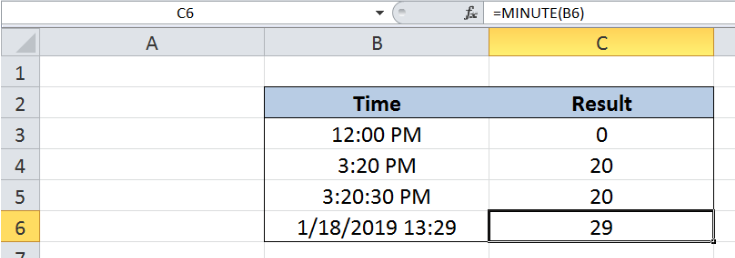Figure 6.  Output: MINUTE function extracting the minute component of time

## Notes:

• In cases where only the date is given, and there’s no time, the MINUTE function returns the value zero “0”
• The extracted value returned by the MINUTE function can be used as input to other functions such as the TIME function

Most of the time, the problem you will need to solve will be more complex than a simple application of a formula or function. If you want to save hours of research and frustration, try our live Excelchat service! Our Excel Experts are available 24/7 to answer any Excel question you may have. We guarantee a connection within 30 seconds and a customized solution within 20 minutes.

### Did this post not answer your question? Get a solution from connecting with the expert.Another blog reader asked this question today on Excelchat:## Subscribe to Excelchat.coAnother blog reader asked this question today on Excelchat: